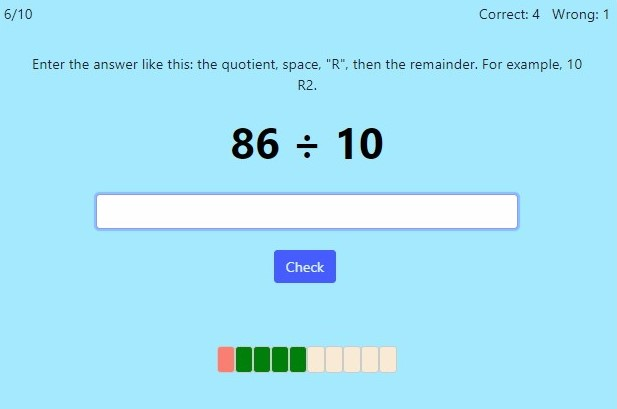MM Practice
×
Multiplication
Division
Place Value
Fractions & Decimals
Measurement
Statistics & Probability
Pre-Algebra
Money
Kindergarten
Geometry

# Division With Remainders (Mental Math)Online practice for grades 3-4

This script allows you to practice division with remainders, using mental math. In other words, the division problems are based on basic division facts (or on multiplication tables), such as 53 ÷ 8 = 6 R5 or 23 ÷ 4 = 5 R3. You can choose timed or untimed practice, the number of practice problems, and the divisors you'd like to include.

Screenshot:OR
Practice for a set time: min

Allow my comment to be posted on this site